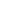# What are pips and how to calculate them correctly?

Concept and features of pips

A pip (a percentage in points) is a basic concept of the forex market. However, even some professional traders cannot understand what a pip is and how to calculate it. Today, we will set the record straight by defining the concept of pips and learning about methods of calculating them.

A pip in the forex market is an indicator that reflects the smallest amount by which the price can change. One pip equals 0.0001. Sometimes, fractions are used. The sole exception is currency pairs that involve JPY. In this case, a pip totals 0.01. The main reason why the ratio of JPY to the leading world currencies differs is that Japan’s legal tender is significantly lower in price.

For example, EUR/USD was trading at 1.3005 at the beginning of the session. By the end of the day, the pair rose to 1.3085, which means that the euro climbed by 80 pips.

Oftentimes, traders identify pips as points in Forex. However, this is not exactly true. In Forex, these definitions are equivalent, but in the stock market, they are not. Thus, 1 pip equals 0.1 USD on the NYSE exchange and reflects the smallest price change. At the same time, 1 point corresponds to 1 USD and equals 100 pips.

Rules on how to calculate pips and apply formulas in practice

Let us explain how to convert a theoretical pip value into a real currency using a few simple formulas. Our calculations will be based on the US dollar and will help us determine the exact amount of profits (losses) during trading.

Calculation 1. This method is used for direct quotes with USD as the base currency. In this case, a Forex pip is the product of the lot size and the smallest price change, divided by the current quote.

Example. We take 1 lot of USD/JPY, the current quote of 87.15, and the smallest change of 0.01. The pip value will be calculated according to the following formula: 100.00 * 0.01/87.15 = 11.47 USD

Calculation 2. This method is used for indirect quotes with USD as a counter currency. In this case, a pip is the product of the lot size and the smallest price change.

Example. We take 1 lot of EUR/USD and the smallest price change of 0.0001. Then, the Forex pip for this currency pair will be 100.0000 * 0.0001 = 10 USD.

Calculation 3. This method is used for cross pairs that do not involve USD. Here, a pip is a product of the lot size, the smallest amount of the price change, and the current price of a major currency pair, divided by the current exchange rate of the pair.

Example. We take 100.0000 lots of EUR/JPY. The smallest change is 0.01, and the current exchange rate of EUR/JPY is 112,46. The major instrument will be EUR/USD with the current price of 1.300. As a result, 1 pip is calculated according to the following formula: 100.0000 *0.01*1.3000*112.50 = 11.56 USD.

Pips play an important role among the basic concepts of the Forex market. We hope that this information will help you understand what a pip is and how it differs from a point. Our examples and calculations will become a useful cheat sheet when converting an abstract definition of a pip to real currency while trading in Forex.

Good luck!